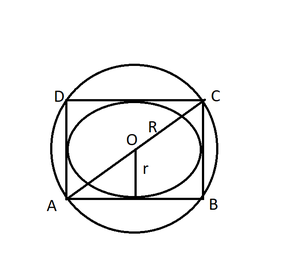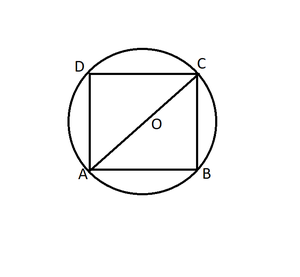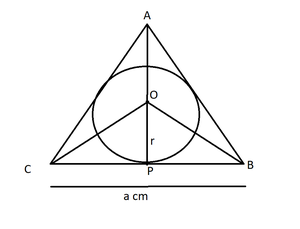Class 10 RD Sharma Solutions – Chapter 15 Areas Related to Circles – Exercise 15.1 | Set 2

• Last Updated : 17 Dec, 2020

Question 11. The radii of two circles are 19 cm and 9 cm respectively. Find the radius and area of the circle which has circumferences is equal to sum of the circumference of two circles.

Solution:

Radius of circle 1 = r1 = 19 cm

Radius of circle 2 = r2 = 9 cm

So, C1 = 2πr1, C2 = 2πr2

C = C1 + C2

2πr = 2πr1 + 2πr2

r = r1 + r

r = 19 + 9

r = 28 cm

Therefore, the radius of the circle = 28 cm

Therefore, area of required circle = πr2

= (22/7) × 28 × 28

= 2464 cm2

Question 12. The area of a circular playground is 22176 m2. Find the cost of fencing this ground at the rate of ₹50 per meter.

Solution:

Area of the circular playground = 22176 m2

Area = πr2

πr2 = 22176

r2 = 22176(7/22)

= 7056

r = 84 m

Circumference of the ground = 2πr

= 2(22/7)84

= 528 m

Cost of fencing 528 m = ₹50 x 528

= ₹26400

Therefore, the cost of fencing the ground = ₹26400.

Question 13. The side of a square is 10 cm. Find the area of the circumscribed and inscribed circles.

Solution:Diagonal of the square = AC = √2 x side

= 10√2 cm

Radius of circumscribed circle = Diagonal/2

R = 5√2cm

R = 7.07cm

Area= πR2

= (22/7) × 7.07 × 7.07

= 157.09 cm

Therefore, the Area of the Circumscribed circle = 157.09 cm

For inscribed circle diameter of circle = side of square = AB

= 10/2

r     = 5 m

Area = πr2

= (22/7) × 5 × 5

= 78.5 cm

Therefore, the area of the circumscribed circle = 157.09 cmand the area of the inscribed circle = 78.5 cm2.

Question 14. If a square is inscribed in a circle, find the ratio of areas of the circle and the square.

Solution:Let side of square AB be x cm which is inscribed in a circle.

Radius of circle (r) = 1/2 (diagonal of square)

= 1/2(a√2)

r = a/√2

Area of the square = a2

Area of the circle = πr2

= π(a2/2)

Ratio of areas = Area of circle:Area of square

= π(a2/2) : a2

= π : 2

Therefore, the ratio of areas of the circle and the square = π : 2

Question 15. The area of circle inscribed in an equilateral triangle is 154 cm2. Find the perimeter of the triangle.

Solution:Area of a Circle = πr2

(22/7) × r2 = 154

r2 = (154 x 7)/22

= 7 × 7

= 49

r = 7 cm

OP is perpendicular bisector of BC (as BP is tangent and it is a equilateral triangle)

BP = ½ x BC

Consider the side of the equilateral triangle be a cm.

In right-angled triangle OPB

OB2 = OP2 + BP2 (By Pythagoras theorem)

OB2 = r2 + (a/2)2    (BP is half of a)

OB2 = 49 + a2/4

OB = √(49 + a2/2)    ….. (1)

AP = (√3/2)a (height of an equilateral triangle)

OA = (√3/2)a – r

Similarly

OB = (√3/2)a – r    …. (2)

From (1) and (2)

Squaring both sides

49 + a2/4 = (3/4)a2 + r2 – √3ar

r = 7

49 + a2/4 = (3/4)a2 + 49 – 7√3a

a2/4- (3/4)a2 = -7√3a

Taking 4 as LCM

(a2 – 3a2) / 4 = -7√3a

-2a2/4 = -7√3a

a = 14√3 cm

Perimeter of equilateral triangle = 3a

= 42√3

Therefore, the perimeter of the triangle = 42(1.73)

= 72.7 cm

My Personal Notes arrow_drop_up